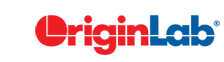# 3.8 FAQ-231 How do I know if my fit result is good?

Last Update: 7/14/2018

When performing nonlinear curve fitting, an iterative procedure is employed that minimizes the reduced chi-square value to obtain the optimal parameter values. The reduced chi-square is obtained by dividing the residual sum of squares (RSS) by the degrees of freedom (DOF). Although this is the quantity that is minimized in the iteration process, this quantity is typically not a good measure to determine the goodness of fit. For example, if the y data is multiplied by a scaling factor, the reduced chi-square will be scaled as well.

A better measure would be the r square value, which is also known as coefficient of detemination. The closer the fit is to the data points, the closer r-square will be to the value of 1. A larger value of r-square does not necessarily mean a better fit because the degress of freedom can also affect the value. Thus if more parameters are introduced, the r-square value will rise, but this does not imply a better fit. The adjusted r-square value accounts for the degrees of freedom and this could be a better measure of the goodness of fit.

Origin reports r-square and adjusted r-square values for linear and polynomial fitting as well as nonlinear fitting, and it also reports reduced chi-square value for nonlinear fitting. The output report sheet can be customized to include, or leave out, any of these quantities.

Statistically speaking, rather than asking whether a particular fit result is good, it is more appropriate to compare two fit results. There are statistical tests that OriginPro provides, to compare the fit results to a single dataset using two different models. Thus one can, for example, compare the fit to decay data with one-term and two-term exponential fitting functions, and determine whether using the two-term fit is justified for the given data. One can also compare two datasets with one fitting function to determine if the two datasets represent the same population, for example.

Origin 9.1 and latter versions provides a tool to rank fitting functions in a category and fit it with the function whose fitted result is the best. Select Analysis: Fitting: Rank Models from Origin menu.

Keywords:quality, goodness, chi square, r square, chi-square, r-square, poor, bad